Joseph M. Mahaffy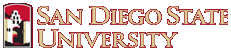Math 531: Partial Differential Equations Spring 2019
|  Course Index  |  Lectures  |  HW Assign  |  WeBWorK  |  Syllabus  |  Miscellaneous  ||  Home

Lectures for Math 531

1. Introduction (4-Panel) - Maple Commands (Maple .mw) - Maple Adoption Discount
2. Ordinary Differential Equations (4-Panel) -
3. Heat Equation - Derivation (4-Panel) -
4. Heat Equation - 3D (4-Panel) -
5. Separation of Variables (4-Panel) - heatjm.m, heat1d (PDF),
6. Separation of Variables B (4-Panel) -
7. Fourier Series (4-Panel) - MatLab: fourier_1.m, gibb_c.m, gibb_d.m, Maple: sawtooth.mw (PDF)
8. String (4-Panel) -
9. Sturm-Liouville Problems (4-Panel) -
10. Sturm-Liouville Problems B (4-Panel) -
11. Sturm-Liouville Problems C (4-Panel) - MatLab: fourier_rob.m, heatjm_rob.m, Maple: robin_ev.mw (PDF)
12. Higher Dimensional PDEs (4-Panel) -
13. Vibrating Circular Membrane (4-Panel) - MatLab: bess_jy0.m, bessel_modes.m, bessel_modesMN.m, besselzero.m, Maple: bessel.mws (PDF), membrane.mws
14. Laplace's on Cylinder (4-Panel) -
15. Nonhomogeneous Problems (4-Panel) -
16. Fourier Transforms (4-Panel) -
17. Fourier TransformsB (4-Panel) - MatLab: heatFT3D.m, heatFTarbf.m, Maple: fourier_heat.mws (PDF)
18. Fourier TransformsC (4-Panel) - MatLab: pde_eg1.m, laplace_eg.m,
19. Method of Characteristics (4-Panel) -

There is a Lab Manual (MATLAB and Maple) version, which will continue to be updated over the semester with detailed information for using MatLab and Maple on your written assignments.

Matlab:

Maple:

Lecture Note Supplements

Chapter 2: Programming Supplement

A class demonstration of Maple and MatLab is given for the one-dimensional heat equation. Links are provided to computer code for Maple (heat1d (PDF)) and MatLab (heatjm.m) for the Heat Equation in one-dimension.

Chapter 3: Programming Supplement

A class demonstration of a Fourier series is given. A link is provided for a MatLab M-file for this Fourier series.

Chapter 5: Programming Supplement

Finding eigenvalues for boundary conditions of the third kind . A link is provided for a Maple file to show how to numerically find eigenvalues of this transcendental equation.

Chapter 7: Programming Supplement

Visualizing vibrating rectangular membranes, we have a link for a Maple file (rect_memb.mws). Also, solutions to Bessel's differential equation are provided using Maple (bessel.mws) and a hyperlink to Maplesoft and others. Solutions to Legendre's differential equation are provided using Maple (legendre.mws).

Below are some MatLab codes and pictures from PDEs provided by Ricardo Carretero.

Matlab:

Maple:

Lectures:

Heat equation in 1D: heat.m.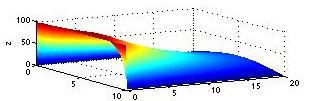PDE integrator in 1D: PDE_integrator.m, wave_plotsurf.m.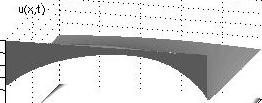Normal modes of wave equation: vibrate.m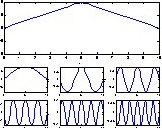Solutions to the linear wave equation: wave_eq.m.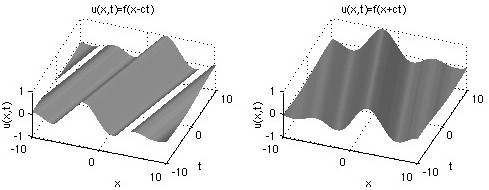D'Alambert solutions to the linear wave equation: ugauss_utzero.m.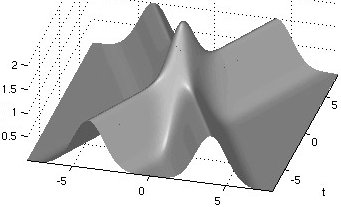Bessel modes: bessel_modes.m, besselzero.m.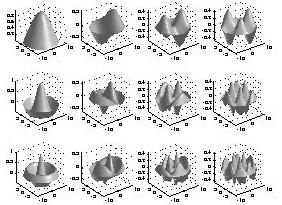For questions concerning the webpage contact Joseph Mahaffy.